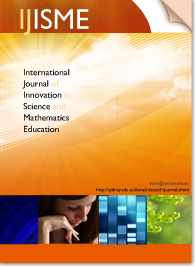# Cognitive analysis on prospective mathematics teachers' reasoning using area and tree diagrams### Resumen

One of the challenges in mathematics education research is to provide a comprehensive description of mathematical activity carried out by university students. Taking this challenge as an objective, this paper analyses the answers of 30 prospective teachers of primary education to a typical mathematics problem on fractions using area and tree diagrams. Theoretical and methodological tools from the onto-semiotic approach to mathematical knowledge and instruction support the cognitive analysis; hence, the underlying complexity of applying the area diagram to express a multiplicative reasoning should be highlighted. However, the structure of the system of practices that have to be carried out to solve the problem in the tree diagram are better aligned with this kind of reasoning. Furthermore, the use of the natural language in order to communicate the answer has been observed as a necessary register. This result lead to a deeper comprehension of the role played by these two types of diagrams and of the mathematical objects that emerge from such representations. As a conclusion, the type of analysis presented here is revealed as a strategic tool for instructors of primary education students to emphasize the importance of meanings negotiation.

Publicación
International Journal of Innovation in Science and Mathematics Education, 27(2), 18-32